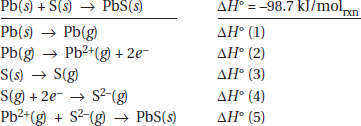# AP Chemistry Practice Test 30

### Test Information12 questions18 minutes

1. Formation of a solution can often be visualized as a three-step process.

Step 1. Solvent molecules are separated from each other to make space for the solute.

Step 2. Solute molecules are separated so they fit into spaces in the solvent

Step 3. Separated solute and solvent are brought together filling in the spaces.

All of the fundamental principles below are important in understanding the formation of solutions except which one?

2. Bromine has a normal boiling point of 59 °C, and iodine boils at 184 °C. The I2 molecule is much larger than Br2 (atomic radii are 114 and 133 pm, respectively). Which is the best reason for the large difference in boiling points?

3.Ethanoic acid (HC2H3O2 or CH3CO2H or CH3COOH) has a much lower vapor pressure than ethanol (CH3CH2OH). What is the most reasonable explanation?

`Questions 4-8 refer to the following information.The synthesis of solid lead(II) sulfide can be expressed in the equation`

5. Which of the reactions above represent the formation of the crystal lattice?

6. If 5.0 g of lead and 1.0 g of sulfur are reacted according to the equation above, what will be left in measurable amounts (more than 1 mg) in the reaction vessel?

7. Which of the following enthalpies will have a negative sign?

8. Which of the following, without regard to sign, will have the same numerical value as the lattice energy, also without regard to sign?

`Questions 9-12 refer to the following information.Questions refer to the cell diagrams and their corresponding E°cell values for the three galvanic cells listed in the table below.Galvanic CellCell DiagramE°cell1Zn | Zn2+(1.0 M) || Pb2+(1.0 M) | Pb0.632Mg | Mg2+(1.0 M) || Pb2+(1.0 M) | Pb2.243Mg | Mg2+(1.0 M) || Zn2+(1.0 M) | Zn?The chemical reaction occurring in the first galvanic cell isZn + Pb2+ → Zn2+ + Pb`

9. What is the standard cell potential for galvanic cell 3 depicted in the table above?

10. What is the chemical reaction under study in galvanic cell 3?

11. If the concentration of Mg2+ is changed from 1.0 M to 0.1 M in the galvanic cells above, what will happen to the observed cell voltages in galvanic cells 2 and 3?

12. Rank Mg, Pb, and Zn from the metal that is easiest to oxidize to the metal that is the most difficult to oxidize.